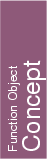A model for this must provide:

Kernel::FT fo.operator() ( Kernel::Sphere_3 s)
returns the squared radius of s.

Kernel::FT
 fo.operator() ( Kernel::Point_3 p, Kernel::Point_3 q, Kernel::Point_3 r, Kernel::Point_3 s)
returns the squared radius of the sphere passing through p, q, r and s.
Precondition: p, q, r and s are not coplanar.

Kernel::FT
 fo.operator() ( Kernel::Point_3 p, Kernel::Point_3 q, Kernel::Point_3 r)
returns the squared radius of the sphere passing through p, q and r, and whose center is in the plane defined by these three points.

Kernel::FT
 fo.operator() ( Kernel::Point_3 p, Kernel::Point_3 q)
returns the squared radius of the smallest circle passing through p, and q, i.e. one fourth of the squared distance between p and q.